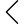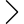13 products found
• s
• m
• l
• xl
• 2xl
• s
• m
• l
• xl
• 2xl
• s
• m
• l
• xl
• 2xl
• s
• m
• l
• xl
• 2xl
• s
• m
• l
• xl
• s
• m
• l
• xl
• s
• m
• l
• xl
• s
• m
• l
• xl
UVP FORESTER HOODED SHIRTS
\$259.00 \$155.00
• s
• m
• l
• xl
• s
• m
• l
• xl
• s
• m
• l
• xl
• s
• m
• l
• xl
• s
• m
• l
• xl
••